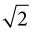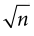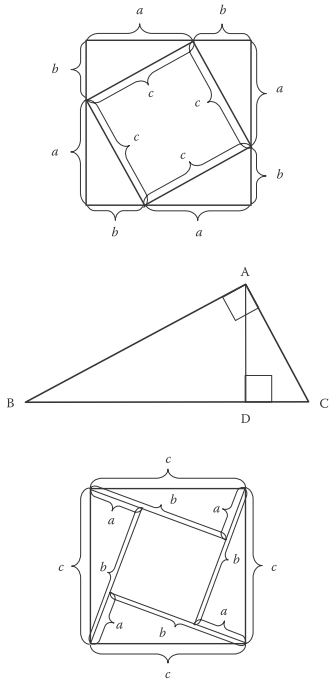Definition: rational number from The Penguin Dictionary of Science

A number that is the ➤quotient of two ➤integers.

Summary Article: Rational and Irrational Numbers
From Computing: A Historical and Technical Perspective
3.1 APPEARANCE OF FRACTIONS

Counting is the simplest and most fundamental operation using numbers. However, just counting was insufficient and inconvenient for people even in ancient civilizations. People at some early stage of their civilizations realized that arithmetic operations such as addition, subtraction, multiplication, and division were necessary and very important. It is probably more than 4000 years ago that people in Egypt, Mesopotamia, and other ancient civilized regions could already manipulate arithmetic operations using their number systems, although it is difficult to specify when and where people first discovered such techniques for calculations.

Among the four arithmetic operations, multiplication and division were difficult compared with addition and subtraction. People invented doubling and halving operations a long time ago. These and similar operations were convenient for their lives, and made multiplication and division easier. Consequently, the fractions 1/2, 1/4, 1/8, 1/16, and so on became commonly used numbers in addition to natural numbers. Furthermore, the use of reciprocals of integers, such as 1/2, 1/3, 1/4, 1/5, 1/6, and so on, also became common and of great importance. The reciprocal of each nonzero natural number is called a unit fraction. They noticed that multiplications of unit fractions by integers were also useful for calculations. In this way, fractions appeared in ancient civilizations .

Ancient Egyptians used special symbols representing fundamental unit fractions and some other fractions (e.g., 2/3). These symbols appeared in several mathematical tablets and papyri found in Egypt . Akhmim wooden tablets and Cairo wooden tablets are two ancient Egyptian documents that contain, for example, descriptions about multiplications by fractions 1/3, 1/7, 1/10, 1/11, and 1/13. The following calculation of fractions also appeared in the tablets:

1/2 + 1/4 + 1/8 + 1/16 + 1/64 + 5/320 = 1

Historians suggest that these tablets were probably inscribed at the beginning of the Egyptian Middle Kingdom around 1950 BC . These are now held in the Egyptian Museum in Cairo.

In the British Museum in London, the Rhind Papyrus (also called Rhind Mathematical Papyrus) is displayed. It is approximately 5 m long and 33 cm wide, one of the oldest existing texts of Egyptian mathematics. A Scottish lawyer, Alexander H. Rhind (1833-1863), purchased it in 1858 in Egypt . It was named the Rhind Papyrus after him. It was copied by a scribe named Ahmes around 1650 BC. In the first paragraph of the papyrus, Ahmes presents that it is copied from an ancient copy made during the 12th dynasty of Upper and Lower Egypt (c. 1985-1795 BC) [3, 8].

The first part of the Rhind Papyrus contains a list of the fractions 2/n for odd n from 3 to 101. The following are examples in the list:

2/3 = 1/2 + 1/6

2/5 = 1/3 + 1/28

2/7 = 1/4 + 1/28

2/9 = 1/6 + 1/18

2/15 = 1/10 + 1/30

2/101 = 1/101 + 1/202 + 1/303 + 1/606

The second and third parts of the Rhind Papyrus consist of geometry problems, and 84 problems with the solutions, respectively. Below is an example in the third part:

Problem 3.1

Let the sum of 2/3 and 1/10 of an unknown quantity be 10. Calculate the unknown quantity.

SOLUTION

Using modern notation, (2/3 + 1/10)x = 10, where x is the unknown quantity. Then x = 300/23.

The Moscow Papyrus (also called Moscow Mathematical Papyrus) is now held in the Puskin State Museum of Fine Arts in Moscow . It is approximately 5.5 m long and 3 ~ 7.7 cm wide, one of the oldest Egyptian mathematical texts in existence. The Moscow Papyrus probably dates to the 11th dynasty of Upper and Lower Egypt (c. 1850 BC). It contains 25 problems of arithmetic, algebra, and geometry. The following are examples of the problems:

Problem 3.2

Let the sum of 1 and a half of an unknown quantity and 4 be 10. Calculate the unknown quantity.

SOLUTION

Using modern notation, (1 + 1/2)x + 4 = 10, where x is the unknown quantity. Then x = 4.

Problem 3.3

Let 1/2 + 1/4 of the square of an unknown quantity be 12. Calculate the unknown quantity.

SOLUTION

Using modern notation, (3/4)(3/4)x2 = 12(3/4), where x is the unknown quantity. Then x = 4.

3.2 RATIONAL NUMBERS

The set of rational numbers is usually denoted by Q, and the elements of this set have the property that they can be represented as a ratio of two integers (hence the name rational). These numbers are, in a sense, measurable, and they include whole integers, fractions, and their together negative counterparts. The set of rational numbers is equivalent to the set of multiplications of unit fractions and integers. That is, we can write

Q = {p/q | p is an integer and 1/q is a unit fraction}

A rational number is one that can be named number, in that we can say seven, three-fourths, 0.3. With this, any rational number can be placed in its exact (measured) position on the real number line (see also Chapter 13). Rational numbers can be represented using decimal notation where the representation is either finite (e.g., two-fifths is 0.40) or infinite, but repeating (e.g., three and two-sevenths is 3.285714285714285714…). Historically the decimal fraction notation (e.g., 3.285714285714285714…) appeared much later than fraction notation (e.g., 3 + 2/7 or 23/7). The use of decimal fraction notation became common in the 16th century (see more in Chapter 10). Since a repeating decimal can be written algebraically as a fraction, it follows that any repeating decimal must be a rational number. Specifically, to prove that any repeating decimal represents a fraction of two integers, let us examine a number x whose decimal representation is of the form i1i2 …. ik .d1d2 …dmabcdabcdabcd …, where the digits i and d represent the integer part, and the nonrepeating portion of x and abcd is the repeating pattern. Algebraically, x = r + y, where r = i1i2 …ikd1d2 …dm/10m, which already is a ratio of two integers, is a rational number. So, we just have to show that the number y = 0.00...0abcdabcdabcd… is also a ratio of two integers. We multiply assign to g the repeating pattern value of abcd, and pick the smallest h such that 10h > abcd; i.e., h is the pattern length. Now, y = abcd/(10h - 1) is a ratio of two integers; hence, it must be rational. Since the set of rational numbers is closed under addition (i.e., a sum of two fractions is a fraction), the original number x is rational.

Let us examine the above formula on the repeating decimal 0.999…. The pattern length here is just one digit. Therefore, we have 9.0/(101 - 1) = 1.0. This is unexpected, because it implies that there are two different representations for the same number, that is, 1. The first is 1.0 and the second is 0.999…. Intuitively, we know that 0.999… is three times 0.333… = 1/3. So the fact that 0.999… = 1.0 is, at least, not counterintuitive. However, it is concerning that the same (rational) number can have two representations. We can prove that 0.999… = 1.0 in another way. Let us solve the following system of equation(s), 0.999… = x, with one equation and one unknown. Algebraically, we can multiply the two sides of an equation by a number and maintain equality. In this case, let the multiplier be 10. So, we have 9.999… = 10x. Now, if we subtract the first equation from the second, we have 9.0 = 9x, so we get our final answer: x = 1.0.

3.3 IRRATIONAL NUMBERS

Pythagoras (c. 570-540 BC) is one of the most famous Greek philosophers and mathematicians. The Pythagorean theorem is a relation among the side lengths of a right triangle. Let a, b, and c be the side lengths of a right triangle, where c is the length of the side opposed to the right angle. The Pythagorean theorem can be expressed as the following equation:

a2 + b2 = c2.

The Pythagorean theorem was probably known much before Pythagoras , but it is said that Pythagoras first proved the theorem. There exist many different proofs of the Pythagorean theorem. It can be proved algebraically or geometrically, e.g., by using the drawings in Figure 3.1.

From the Pythagorean theorem, the Greek mathematicians, probably including Pythagoras, realized that there exist numbers that cannot be measured by a rational number. The most obvious example was the length of the diagonal of a one-by-one square, which isfrom the Pythagorean theorem. More generally, if n is a positive integer and not a square number, thenis not a rational number. The proof of this fact is given in Theaetetus (c. 360 BC) written by the Greek philosopher and mathematician Plato (427-347 BC). They noticed that these numbers cannot be represented by a ratio of two integers. Arabic mathematicians treated these numbers as algebraic objects. Hindu and other mathematicians were also aware of the existence of such numbers and that they are much more mysterious than the rational numbers, but none of the early mathematicians grasped the full meaning and magnitude of this set.

Pythagoras wanted to believe that all numbers were rational (could be written as a fraction or be measured). Hippasus (fifth century BC), who was Pythagoras's student, actually showed that the square root of 2 cannot be a ratio of two integers. It is believed that he used a geometrical (and not an algebraic) argument. Pythagoras was caught in a dilemma: what to do with a number that was not rational, i.e., irrational. Despite Hippasus's proof, he would not accept the existence of irrational numbers. Since Pythagoras could not disprove the existence of irrational numbers, he had Hippasus thrown overboard and drowned! An unfortunate footnote to this is thatis often called Pythagoras's constant.FIGURE 3.1 Three different proofs of the Pythagorean theorem.

Let us present a simple (non-constructive ) proof that there can exist no rational number x whose square is 2. Suppose such a number existed, i.e., x = m/n, where n is not 0, m/n is simplified to the lowest terms (i.e., m/n is an irreducible fraction), and x2 = 2. It follows that (mm)/(nn) = 2. Therefore, both m and n cannot be even numbers—at least one of them must be odd since m/n is irreducible. From the equation, we can derive n2 = 2m2, which is an even number. This implies that m must be an even number, e.g., m = 2k, where k is one-half of m. Now, let us substitute for m. We have (mm)/(nn) = 2 = (2k)2/(nn) = 4k2/(nn). So, 2nn = 4k2, or equivalently, nn = 2k2. Therefore, nn must be even, which makes n even. But both m and n could not be even (since m/n is irreducible), and so we have a contradiction. This means that our assumption thatis a ratio of two integers (rational number) is not correct.

While the majority of tasks of daily life involve rational numbers, the irrational numbers are just as important, and as will be shown later, there are much more irrational numbers than we can count (see Chapters 13 and 14).

REFERENCES
• 1. Wikipedia, Real Number, http://en.wikipedia.org/wiki/Real.
• 2. Wierstrass's Construction of the Irrational Number, Math Semester, 58, 47-58, 2011.
• 3. History of Mathematics (Vol. II), Dover Publications New York, 1958.
• 4. Mathworld, http://mathworld.wolfram.com/IrrationalNumber.html.
• 5. Wikipedia, Irrational Number, http://en.wikipedia.org/wiki/Irrational_number#cite_note-28.
• 6. ; Philosophies of Mathematics, Blackwell, 2002.
• 7. Wikipedia, Fraction, http://en.wikipedia.org/wiki/Fraction.
• 8. Wikipedia, Rhind Papyrus, http://en.wikipedia.org/wiki/Rhind_mathemati-cal_Papyrus.
• 9. Wikipedia, Moscow Papyrus, http://en.wikipedia.org/wiki/Moscow_Papyrus.
• 10. Wikipedia, Akhmim Wooden Tablets, http://en.wikipedia.org/wiki/Akhmim_wooden_tablets.
• 11. The Universal History of Computing, John Wiley & Sons New York, 1981.
• 12. ; The Rhind Mathematical Papyrus: Ancient Egyptian Text, British Museum Publications London, 1987.
• 13. Mathematics in Egyptian Papyri, http://www-history-mcs.st-andrews.ac.uk/HistTopics/Egyptian_papyri.html.
• 14. Geometry and Algebra in Ancient Civilization, Springer-Verlag Berlin, 1983.
•© 2014 by Taylor & Francis Group, LLC

### Related Articles

##### Full text Article Rational Numbers
Bloomsbury Guide to Human Thought

A rational number, in mathematics, is one which can be represented by a fraction, the ratio of two integers (the one which does the...

See more from Credo